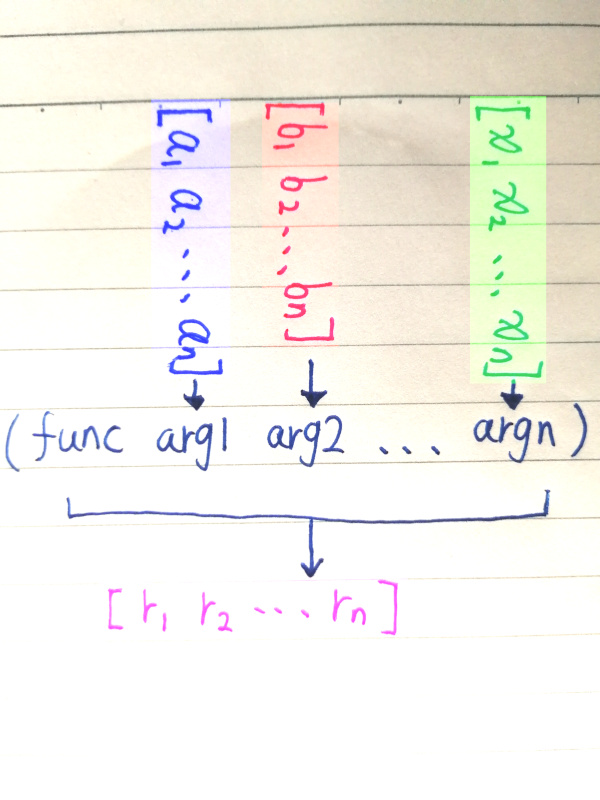# SICP Goodness - Stream (III)

Doing some exercisesDo you think Computer Science equals building websites and mobile apps?

Are you feeling that you are doing repetitive and not so intelligent work?

Are you feeling a bit sick about reading manuals and copy-pasting code and keep poking around until it works all day long?

Do you want to understand the soul of Computer Science?

If yes, read SICP!!!

To test our knowledge, let’s do some exercises from the book.

Exercise 3.50: Complete the following definition, which generalizes stream-map to allow procedures that take multiple arguments, analogous to map in 2.2.1, Footnote 12.

``````(define (stream-map proc . argstreams)
(if (⟨??⟩ (car argstreams))
the-empty-stream
(⟨??⟩
(apply proc (map ⟨??⟩ argstreams))
(apply stream-map
(cons proc
(map ⟨??⟩
argstreams))))))
``````

First of all, let’s look at footnote 12:

Scheme standardly provides a map procedure that is more general than the one described here. This more general map takes a procedure of n arguments, together with n lists, and applies the procedure to all the first elements of the lists, all the second elements of the lists, and so on, returning a list of the results. For example:

``````(map +
(list 1 2 3)
(list 40 50 60)
(list 700 800 900))
(741 852 963)

(map (lambda (x y) (+ x (* 2 y)))
(list 1 2 3)
(list 4 5 6))
(9 12 15)
``````

Let’s draw a picture to illustrate this:This is like, for each parameter of the function, you feed in a list of values. The result of the map is just a list of outcomes from those executions.

Having said that, let me present the anwser of the exercise.

``````(define (stream-map proc . argstreams)
(if (null? (car argstreams))
'()
(cons-stream
(apply proc (map stream-car argstreams))
(apply stream-map
(cons proc (map stream-cdr argstreams))))))
``````

There are several things that you have to understand first in order to understand what it is all about.

First, the dot in the function definition. This basically means put everything coming after the dot in a list called argstreams. Let’s try it out:

``````(define (try-out arg1 . arglist)
(display arglist))

(try-out "first-arg" 1 2 3 4 5)
;(1 2 3 4 5)
``````

The next thing to understand is `apply`. If you know javascript, this should be very familiar to you. Basically, `apply` is used to call a function. Sometimes you have all the arguments as a list, and when you call the function you want to somehow using the list directly. For example:

``````(define params (list 1 2 3))

params
;Value: (1 2 3)

(apply + params)
;Value: 6
``````

Now you should be able to understand the answer with no problems.

The next exercise Exercise 3.51 is tricker than I thought if you want to fully understand what is going on, so I will take time to explain things step by step in next post. Stay tuned.